## Acta Scientific Applied Physics

#### Research Article Volume 3 Issue 3

Structure and Evolution of the 1D and 2D Solitons on Shallow Fluid with Varying Relief of Bottom

Vasily Yu Belashov1*, Elena S Belashova2 and Oleg A Kharshiladze3

1Kazan Federal University, Russia
2Kazan National Research Technical University named after A.N. Tupolev - KAI, Russia
3Iv. Javaxishvilis Tbilisi State University, Georgia

*Corresponding Author: Vasily Yu Belashov, Kazan Federal University, Russia.

Received: January 21, 2021; Published: February 27, 2021

#### Abstract

The results of numerical study of evolution of the solitons of gravity and gravity-capillary waves on surface of shallow fluid when the characteristic wavelength is essentially greater then depth, λ > H , are presented for the cases when dispersive parameter is a function of time and spatial coordinates, β = β(t, x, y) . This corresponds to the problems when the relief of bottom is changed in time and space. We use both one-dimensional approach (the equations of the KdV-class) and also two-dimensional description (the equations of the KP-class) where it is necessary.

Keywords: Structure; Shallow Fluid; KdV-class

Basic equations and general properties of solutions

Let us consider the models of the Korteweg – de Vries (KdV) and Kadomtsev – Petviashvili (KP) equations in their application to hydrodynamics, namely, to description of the gravity waves on the surface of an ideal incompressible fluid of small (compared to wavelength) depth. In this case, the generalized density and velocity of “sound” in the general set of the hydrodynamic equations  acquire the sense of fluid depth, H, and velocity c=√gH,the term gH2/2 plays the role of the pressure, this corresponds to the effective adiabatic index γ = 2 . Then the Boussinesq equations take the form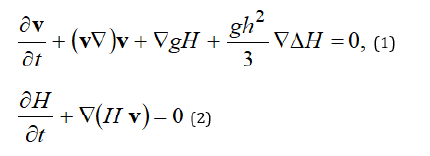(h=const is the depth of the fluid). It is easy to add into these equations the terms associated with the capillary effects. Assuming that the curvature of the surface is not too large and the additional pressure to the fluid caused by the surface tension is defined by the Laplace formula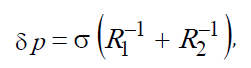Where σ is the surface tension coefficient, R1 and R2 are the main curvature radii, we can write δp = −σΔη where η(x, y,t ) is the surface function (the value of η is sufficiently small). Changing ρgh in (1) by ρgH+ δp (ρ is the fluid density) we obtain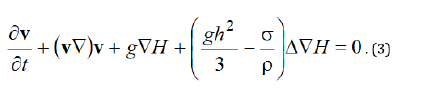Equations (2), (3) are the Boussinesq equations taking into accounting the capillary effects . Change of the factor at the dispersive term in the dispersion relation in its standard form  leads to the change of the dispersion equation, and, instead of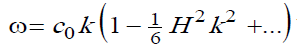we have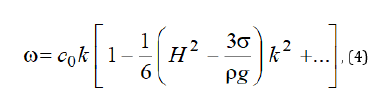Where Co = √gH. In this case the dispersive factor is defined by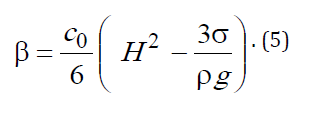Using furthermore the results of  we transform (1) and (2) to the form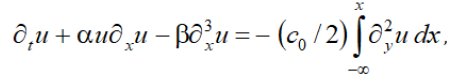i.e. obtain the KP equation for the gravity-capillary waves on shallow fluid. Note that for sufficiently large σ>1/3δpH2 the dispersive parameter changes its sign that involves the qualitative change of the character of the evolution and the form of the solutions .

Consider now in more detail the following interesting case. Often there are the cases when the factor β is unusually small. As it follows from (5) β = 0 at H = (3σ / ρg )1/ 2 ≈ 0.48cm( for pure water). However β = 0 does not mean that there is no dispersion in medium. It simply means that in this case the next term in the Taylor expansion in k of the full dispersion relation must be taking into account. In this case, the corresponding additional term proportional to the next odd derivative appears in the equation. This generalization leads to the KP equation which can be written asWhere the coefficients are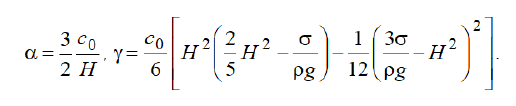Numerical integration of (6) using the methods based on implicit and explicit difference schemes [1,3] enables us to investigate the structure of the one-dimensional (1D) and two-dimensional (2D) solitons on shallow fluid in the case of anomalously weak dispersion. We have found that the qualitative form of the solutions depends significantly on the value of parameter ε = (β /V ) (−V /γ ) 1/2<< 2 where V is the soliton’s velocity in the reference frame moving along the x-axis with the phase velocity ε = 0. In 1D case at the structure of propagating solitons does not differ qualitatively from the structure of solitons of the usual KdV equation (see ), and in 2D case – from the structure of the algebraic KP-solitons [1,3]. Such solitons on the surface of fluid have negative polarity (the hollow solitons). When ε >0, for example, in the case of the increasing fluid depth starting from the depth H = (3σ/ ρg )1/ 2 , the structure of the solitons radically changes: by remaining to decay from their maximum to zero in the transverse direction as before, now their sign varies along the direction of their propagation (at this, the amplitude of the 2D solitons falls from maximum to zero in the transverse direction as before). At ε → 2 the number of the oscillations in the tails increases and the solitons become similar to 1D and 2D high-frequency trains, respectively, i.e. envelope solitons1. Note that a similar structure is typical also for solitons of internal gravity waves, which were considered in detail in [1,4,5].

Let us consider now, separately for 1D and 2D cases, some our results of numerical simulation of the soliton dynamics on surface of shallow fluid which describes by the standard KdV and KP equations (equation (6) with γ = 0 ) when factor β is a function of space coordinates and time.

Structure and evolution of 1D solitons of gravity and gravitycapillary waves with varying relief of bottom

At first, let us consider the evolution of the 1D solitons in the framework of model (6) with γ = 0 and right-hand side being equal to zero (the KdV equation):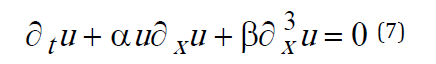on surface of a fluid with varying in time and space dispersive parameter β = β(t ,x). Such situation can take place, for example, in the problems on propagation of the gravity and gravity-capillary waves on surface of shallow fluid  when β = c0 H2/6 and β = (c0/6 )[H2 − 3σ / ρg] , respectively (see above). In these cases, if H =H (t ,x), the dispersive parameter becomes also the function of the x coordinate and time.

In [1,4] it was shown that the solutions of the KDV equation at β=const in dependence on value of β are divided into two classes: at β < u0 (0, x) l / 12 (where l is the characteristic wavelength of the initial disturbance) they have soliton character, in the opposite case the solutions are the wave packets with asymptotes being proportional to the derivative of the Airy function (see also ). In these cases, the KdV equation can be integrated analytically by the inverse scattering transform (IST) method. But, even in 1D case, if β = β(t ,x), this approach is impossible principally, it is necessary to resort to a numerical simulation in the conforming problems.

Let us formulate the problem of numerical simulation of the KdV equation with β = β(t ,x) and consider some results of our numerical experiments on study of structure and evolution of the solitary waves on surface of shallow fluid.

To solve the initial problem for the KdV equation (7) with variable dispersion we used an implicit difference scheme  with O(τ2 , h4 ) approximation. Initial conditions were chosen in form of the solitary disturbance,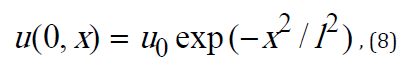and in form of a “smoothed step”: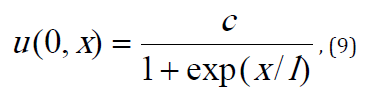With different values of parameters u0, l and с, which were defined by the convenience of numerical calculation for specific sizes of the numerical integration area. The zero conditions on boundaries of the computation region were imposed, and simulation has been conducted for a few types of model types of function β (see figure 1 and 2) when for t < tcr β = β0 = const, and for t ≥ tcr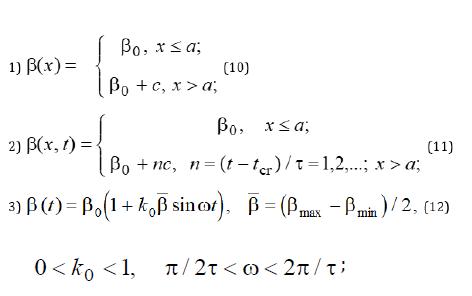Where a and c are constants. In terms of the problem of the wave propagation on surface of the shallow water that accordingly means that on reaching tcr we have: 1) sudden "breaking up of the bottom", 2) gradual "changing of height" of the bottom area, and 3) "bottom oscillation" with time.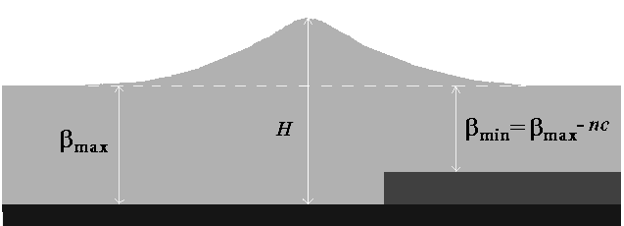Figure 1: Dependence β = β(t ,x) of type of “step”, models (10) and (11).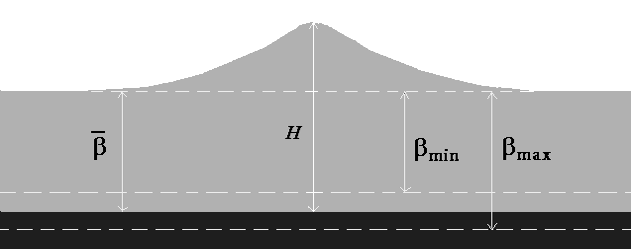Figure 2: Dependence β = β(t ,x) of type of “bottom oscillations”, model (12).

Consider briefly some results of numerical simulation for two types initial conditions and different kinds of model function β = β(t ,x).

In the first series of numerical experiments we investigated of the evolution of the initial disturbance in form of the solitary soliton-like pulse (8) for the models with spasmodic change of dispersion [models of bottom of type of “step” (10) and (11)] with values of parameter а corresponding (at t = 0 ) to the position of the “break” behind and ahead soliton, and values с < 0 (“negative" step) and с > 0 (“positive” step). The obtained results showed that in all cases the deformation of initial pulse occurs with time. If the step is located behind the soliton, that in both cases, c<0 and c>0, the waving tail which is not associated with the main maximum of the outgoing forward main pulse is formed, and its evolution is entirely determined by the value β in its location. In case when at t = 0 the “step” is located ahead front of initial pulse, at с > 0 in the model (11) a steep front is formed quite quickly, that leads to the overturning of the wave with time. At с > 0, the destruction of the soliton can be observed (Figure 3), which occurs due to the fact that in the region of localization of its front, the relative role of nonlinear effects falls due to the increase of the dispersive parameter here, and dispersive effects prevail.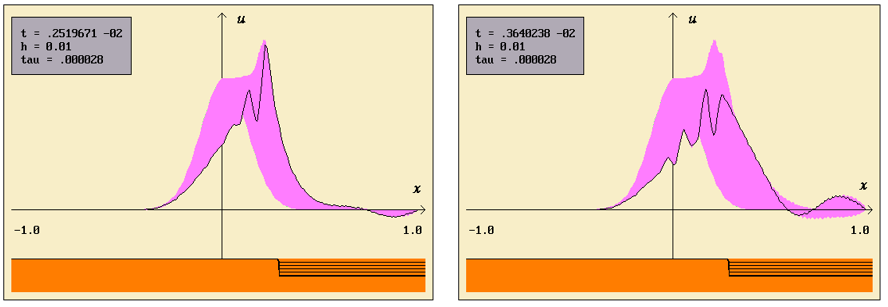Figure 3: Evolution of the KdV soliton in the model (11) with с< 0.

The second series of numerical experiments was devoted to study of the evolution of the initial disturbance of type (9) for the models of “bottom” (10), (11) at different values of parameters а and с.

Figure 4 shows the result of numerical simulation of the evolution of initial disturbance (9) for the model of “bottom” in the form of positive step in the case when “break” is located directly under the region of the disturbance front of the fluid surface. It is seen that due to the fact that the development of perturbations occurs mainly in the region where the value of the dispersion parameter corresponds to multisoliton solution of the KdV equation [1,2], solitary disturbance propagates with the development of highfrequency oscillatory structure behind the shock front, and in the region of the soliton "tail", where dispersive effects dominate over the nonlinear ones, the high-frequency train of oscillations decays rather quickly to zero and it is limited in the region x < 0 .Figure 4: Evolution of “step” (9) in the model (10) with с>0: a − t ≈ 0.25; b − t ≈ 0.5.

Figure 5 shows the example of the results of simulation of the evolution of initial disturbance in the form of the “smoothed step” (9) in case when the break of the “bottom” is negative and located in front of the localization region of the fluid surface disturbance. It can be seen that in this case, the front of the disturbance becomes more gentle with time, the oscillatory soliton structure in the front region is not formed, but the development of low-frequency oscillations behind the main maximum occurs. This result is easily explained within the framework of the similarity principle for the KdV equation : the evolution of the "tail" of the initial disturbance occurs in the region of small values of the dispersive parameter, whereas in the front region, where the dispersion is relatively large, the formation of a shock wave does not occur.

As for the third law of change of β (harmonic oscillations of the parameter β with time on all x-axis), a series of numerical experiments for various k0 = const at variable frequency ω [see law of change (12)] showed that at some values of ω the stationary (locally) standing waves can be formed, in other cases it is possible formation of the stationary periodic wave structures, in intermediate cases a chaotic regime is usually realized.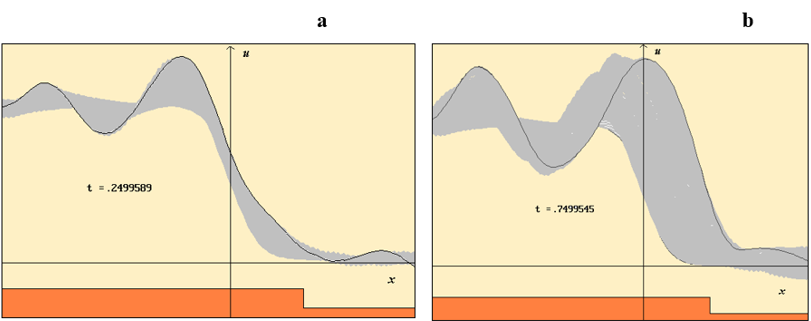Figure 5: Evolution of “step” (9) in the model (10) with с<0: a − t ≈ 0.25; b − t ≈ 0.75.

Structure and evolution of 2D solitons of gravity and gravitycapillary waves with varying relief of bottom

Let us now consider the problem of evolution of the 2D solitons in the framework of the standard KP equation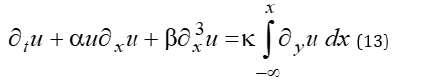With varying in time and space dispersive parameter β = β (t , x, y) . This situation can take place in the problems on propagation of gravity and gravity-capillary waves on surface of shallow fluid  when the fluid depth is the function of the spatial coordinates and time H = H(t , x, y) .

Here, situation is the same as for 1D model of the KdV equation described above take place: if the analytical solutions of the KP equation are known that in case β = β (t,r) the dispersion term of equation becomes quasi-linear that the model being not exactly integrable (the IST method is not applicable) .

The problem of numerical simulation of the KP equation with O(τ2 , h4 ) is formulated analogously the problem for the KdV equation (see previous section). To solve the initial problem for the KP equation (13) with variable dispersion (varying relief of the bottom) we used an implicit difference scheme  with O(τ2 , h4 ) approximation. Initial conditions was chosen in form of the exact 2D one-soliton solution of the KP equation , the complete absorption conditions on boundaries of the computation region [1,3] were imposed, and simulation has been conducted for the same types of model function β as for the KdV equation [see formulae (10)-(12)].

Consider basic results of the numerical experiments on the investigation of the structure and the evolution of 2D solitary waves on the fluid surface with variable dispersion.

The first series of numerical experiments have been aimed at study of the soliton dynamics under spasmodic character of the dispersion change (function β = β(t, x, y) has form of the "step"). At first, we investigated the evolution of initial pulse in case when at tcr the spasmodic change of β has a place behind soliton ["negative" step when с < 0 in formulae (10), (11)]. At this, the dependence of the spatial structure of solution on the parameter a value in models (10) and (11) was studied. The obtained results (see an example in figure 6) showed that in all cases the evolution leads to the formation of waving tail which is not connected with soliton going away and caused only by local influence of sudden change of the “relief” β = β(t, x) . Consequently, the formation of oscillatory structure is connected not so much with decreasing of a role of the dispersion effects behind soliton as with the spasmodic changing of β in space.Figure 6: Solution of eq. (13) for the dispersion law (10) with a=5.0, c=−0.0038 at t=0.6.

In the next series of numerical simulation, we considered the evolution of a 2D soliton in case when the sudden change of the dispersion parameter has a place directly under or in front of an initial pulse ("negative" step). An example of the results of that series is shown in figure 7. From the analysis of the results of whole of the series one can see that for such character of the relief of function β the disturbance caused by sudden change of the dispersive parameter has also a local character, i.e. it doesn't propagate together with the going away soliton. But, unlike the cases considered in the first series of simulation, the asymptotes of leaving soliton become oscillating (in any case in the time limits of numerical experiment), besides, against a background of the longwave oscillations of the waving tail we can also see the appearance of the wave fluctuations. The effects noted may be interpreted as a result of those that for the areas of the wave surface with different values of local wave number kx the value of the dispersive effects is different. As a result, the intensity of the phase mixing of the Fourier-harmonics within (x, y)-region varies with the coordinates and, therefore, it reacts differently to the nonlinear generation of the harmonics with various (in particular, large) wavenumbers kx.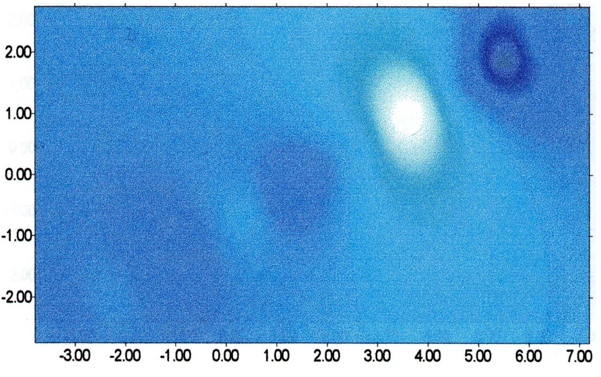Figure 7: Solution of eq. (13) for the dispersion law (11) with a=4.0, c=−0.0038 at t=0.6.

In the third series of the experiments with dispersive parameter changing with the laws (10) and (11) we considered the cases of "positive" step [ c > 0 in formulae (10) and (11)] being both in front of and behind of initial pulse for the wide diapason of values of parameter а. The examples of the most interesting results are shown in figure 8. One can see that when "positive" step is far in front of maximum of the function u (0, x, y) the soliton evolution on the initial stage does not practically differ qualitatively from that for β = const (Figure 8,a), but in the future the evolution character is defined by presence of the step, namely the processes, caused by the same causes which have been noted for the results of the second series of numerical simulation, begin to be developed (figure 8,b). As we can see in the figure, the appreciable change of the soliton structure which can lead to wave falling is observed owing to intensive generation of the harmonics with big kx in the soliton front region, even for rather small height of the step (i.e. even if the value of parameter c in formulae (10), (11) is rather small). Thus, as it follows from the results of this series, the disturbance of the propagating 2D soliton caused by sudden change in time and space of the dispersive parameter with с > 0 has also local character.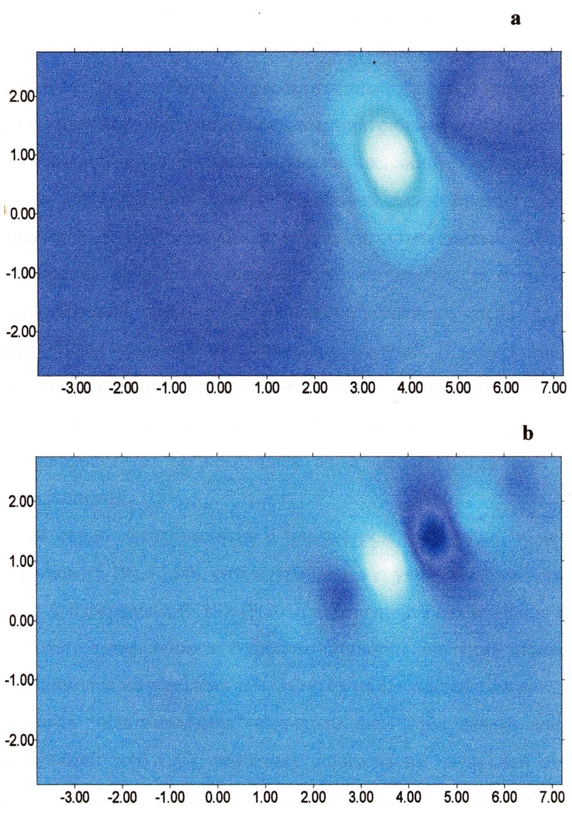Figure 8: Evolution of soliton of eq. (13) for the dispersion law (11) with a=5.0, c=0.0038: (a) t=0.6, (b) t=0.8.

As to the second law of the β change (model (12) − harmonic oscillation of the parameter β with time on the whole (x, y)-plane), the series of numerical simulation for different k0 = const and variable frequency ω [see law (12)] showed that for some values of ω the stationary (locally) standing waves can be formed, in other cases the formation of the stationary periodical wave structures is possible, and in the intermediate cases a chaotic regime is usually realized.

In the experiments made for different values of the parameter k0 and ω = const , we found that the stable (in any case in the limits of the numerical computation time) solutions can be formed only for k0 ≤ β0 in formula (12), and the solutions are unstable in another cases. An example of evolution of the 2D soliton when its structure along the x and y axes acquires the wave character and the amplitude of its maximum decreases with time is shown in figure 9.Figure 9: Evolution of 2D soliton of eq. (13) at t =0.4, 1.2, 2.0.

Summing up the above, one can note that the numerical simulation of evolution of the 2D solitons describing by the model of the KP equation with β = β(t, x, y) enabled us to found different types stable and unstable solutions including the solution of the mixed "soliton non-soliton" type for various character of the dispersion change in time and space.

Obtained results open the new perspectives in the investigation of a number applied problems of the dynamics of the nonone- dimensional nonlinear waves in the specific physical media, including upper atmosphere (ionosphere), magnetosphere and in a plasma [1,3-5].

The work is performed according to the Russian Government Program of Competitive Growth of Kazan Federal University. This work was also supported by the Shota Rustaveli National Science Foundation (SRNF), grant no. FR17 252.

#### Conclusions

In the paper the results of numerical study of evolution of the solitons of gravity and gravity-capillary waves on surface of shallow fluid when the characteristic wavelength is essentially greater then depth, β = β(t, x, y) , were presented for the cases when dispersive parameter is a function of time and spatial coordinates, β = β(t, x, y) . This corresponds to the problems when the relief of bottom is changed in time and space. We have considered three cases of variable dispersion when the sudden "breaking up of the bottom", the gradual "changing of height" of the bottom area, and the "bottom oscillation" with time take place. To solve the problem, we used both 1D approach (the equations of the KdV-class) and also 2D description (the equations of the KP-class). For all cases the numerical solutions of the problem in 1D and 2D geometry were presented. It was noted that the approach realized can be useful also in other applications of nonlinear wave theory such as dynamics of 1D and multidimensional solitary waves in other specific physical media, including upper atmosphere (ionosphere), magnetosphere and in a plasma (see, for example, the papers [6-8]).

#### Bibliography

1. Belashov VYu and Vladimirov SV. “Solitary Waves in Dispersive Complex Media. Theory, Simulation, Applications”. Springer-Verlag GmbH and Co., (2005): 303.
2. Karpman VI. “Non-linear waves in dispersive media”. Pergamon, Oxford, (1975): 175.
3. Belashov VYu. “The KP equation and its generalizations”. Theory, applications. NEISRI FEB RAS, Magadan (1997): 162.
4. Belashov VYu and Belashova ES. “Solitons: theory, simulation, applications”. Kazan Federal University, Kazan (2016): 270.
5. Belashov VYu., et al. “Nonlinear wave structures of the soliton and vortex types in complex continuous media: theory, simulation, applications”. Lecture Notes of TICMI 18 (2017): 90.
6. Belashov VYu., et al. “Structure and evolution of IGW and TID in regions with sharp gradients of the ionospheric parameters”. Journal of Geophysical Research 112 (2007): A07302.
7. Belashov VYu and Belashova ES. “Dynamics of IGW and traveling ionospheric disturbances in regions with sharp gradients of the ionospheric parameters”. Advances in Space Research2 (2015): 333-340.
8. Popel SI., et al. “Evolution of perturbations in a dusty plasma with a variable charge”. Plasma Physics5 (2001): 1497-1504.

#### Citation

Citation: Vasily Yu Belashov., et al. “Structure and Evolution of the 1D and 2D Solitons on Shallow Fluid with Varying Relief of Bottom". Acta Scientific Applied Physics 2.3 (2021): 14-21.

#### Copyright

Copyright: © 2021 Vasily Yu Belashov., et al. This is an open-access article distributed under the terms of the Creative Commons Attribution License, which permits unrestricted use, distribution, and reproduction in any medium, provided the original author and source are credited.

## Metrics

Acceptance rate35%
Acceptance to publication20-30 days

## News and Events

• Certification for Review
Acta Scientific certifies the Editors/reviewers for their review done towards the assigned articles of the respective journals.
• Submission Timeline for Upcoming Issue
The last date for submission of articles for regular Issues is June 20, 2021.
• Publication Certificate
Authors will be issued a "Publication Certificate" as a mark of appreciation for publishing their work.
• Best Article of the Issue
The Editors will elect one Best Article after each issue release. The authors of this article will be provided with a certificate of “Best Article of the Issue”.
• Welcoming Article Submission
Acta Scientific delightfully welcomes active researchers for submission of articles towards the upcoming issue of respective journals.